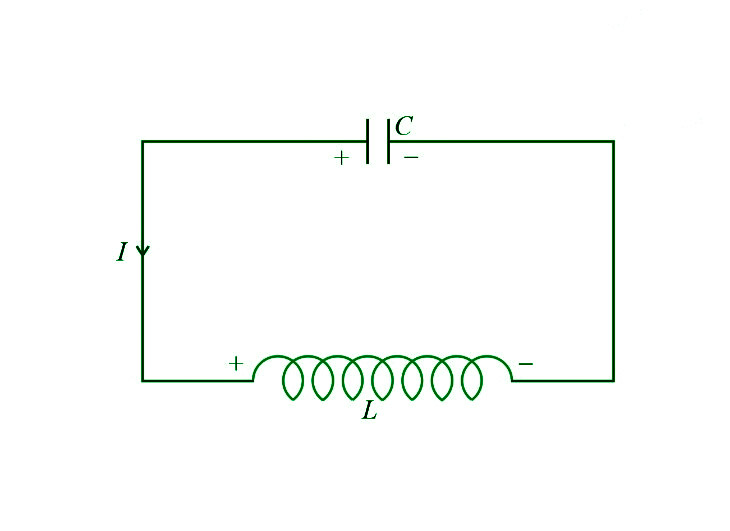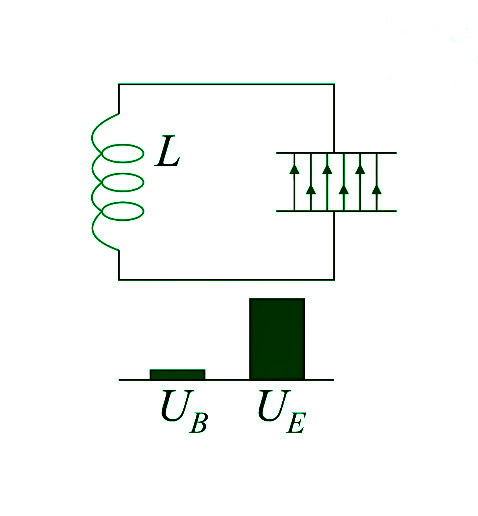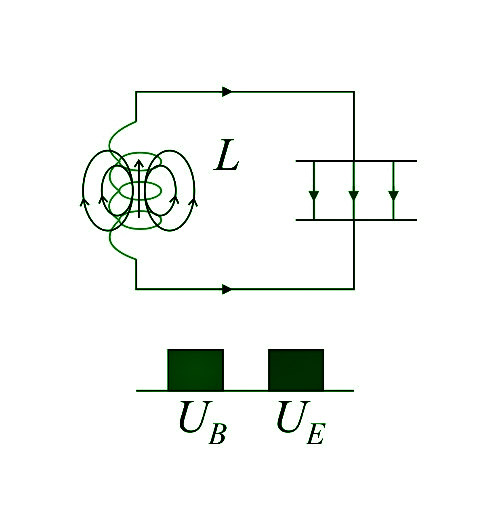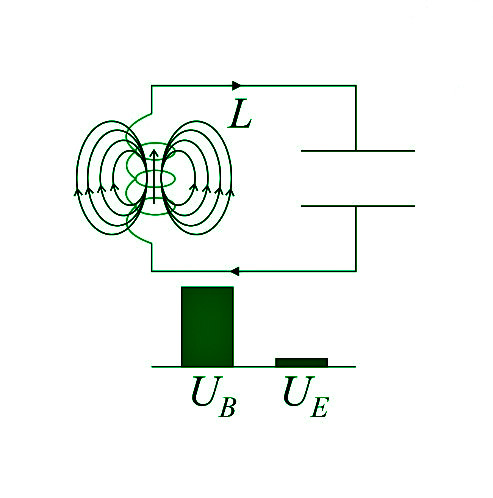GFG App
Open AppBrowser
Continue

The Difference between the Direct and Alternating current is that the direct current (DC), travels only in one direction while the alternating current (AC) is an electric current that alternates direction on occasion and alters its amplitude continuously over time. Alternating current is the type of electricity supplied to businesses and residences, as well as the type of energy utilised by consumers when they plug in kitchen appliances, televisions, fans, and electric lamps to a wall socket.

However, a flashlight’s battery cell is a typical source of DC power. When modifying current or voltage, the acronyms AC and DC are frequently used to denote merely alternating and direct. In most electric power circuits, the most common waveform of alternating current is a sine wave, whose positive half-period correlates to the positive current direction and vice versa. Different waveforms, such as triangle waves or square waves, are utilised in particular applications, such as guitar amplifiers. Alternating current also includes audio and radio signals sent through electrical cables. Information like sound (audio) or pictures (video) is occasionally conveyed via modulation of an AC carrier signal in these forms of alternating current. The frequency of these currents is usually higher than that of power transmission currents.

### What are LC Oscillations?

The LC Oscillator employs a tank circuit (comprising an inductor and a capacitor) to provide the necessary positive feedback to keep oscillations in a circuit going.

A charged capacitor (C) is linked to an uncharged inductor in this circuit, as the name indicates (L).LC Oscillator

A fully charged capacitor and a totally de-energized inductor will be used in this circuit. This inductor’s resistance must be as low as feasible (ideally zero).

Consider this: if a charged capacitor is linked to a resistor, the resistance will consume the energy from the capacitor, and the current flow will ultimately come to a standstill.

However, in this situation, the capacitor (which stores electrical energy) is linked to a low-resistance inductor (which stores magnetic energy). As a result, as the inductor begins to absorb energy from the capacitor, it becomes charged, and its energy rises, causing the capacitor to discharge. When the inductor is completely charged, the capacitor loses all of its energy, and the inductor begins to charge the capacitor using the energy it has stored. The energy is transferred from the capacitor to the inductor and then back to the capacitor. LC Oscillations are the continual flow of energy from one device to another.

### Equations of Energy Stored in a Capacitor and an Inductor

• Energy Stored in a Capacitor

The capacitor is an electric charge and energy storage device. The voltage difference (V) applied between the ends of a capacitor is exactly proportional to the quantity of charge stored (q). As a result, the charge stored in a capacitor has the following equation:

q = CV

The capacitance of the capacitor is denoted by C.

The change in electric potential energy is now calculated as follows:

dU = q (dV)

dU = CV dV

Integrating the equation as,

U0 dU = ∫V0 CVdV

U = 1/2 CV2 = 1/2 qV = q2 / 2C

These are the formulas for calculating the amount of energy stored in a capacitor.

• Energy Stored in an Inductor

The inductor is a device that stores energy in a magnetic field when a current is passed through it. The change in electric current with respect to time is exactly proportional to the potential difference (V) across the ends of an inductor (di/dt).

V = –L di/dt

The inductor’s inductance is denoted by the letter L. The potential energy stored in the inductor will now shift as follows:

dU = V i(dt)

dU = L i(di)

Integrating the equation as,

U0 dU = ∫i0 L idi

U = 1/2 L i2

This is the formula for calculating the energy stored in an inductor.

### Working of an LC Oscillator

When a fully powered capacitor is linked to a de-energized inductor, the capacitor receives all of the circuit’s energy, while the inductor receives none. Let’s call the energy stored in the capacitor (electrical energy) UE and the magnetic energy stored in the inductor (UB).• Current flows from the capacitor to the inductor, energising the inductor and discharging the capacitor. The inductor’s energy begins to rise, while the capacitor’s energy begins to fall. The circuit’s present state is depicted in this diagram. The bars below the circuit diagram show that half of the energy stored in an inductor is equivalent to half of the energy stored in a capacitor at this point in time, indicating that the capacitor has transferred half of its energy to the inductor.• All of the capacitor’s energy will now be transmitted to the inductor as soon as the capacitor is entirely drained. As a result, a significant amount of electric energy is transformed to magnetic energy.• Because the capacitor has been fully drained and the inductor has been fully energised, the inductor will now begin to charge the capacitor in the same direction as the current. The present state of the circuit is depicted in the fourth diagram. As a result, half of the inductor’s energy has been transferred to the capacitor.• Finally, the capacitor will be fully charged again, and the inductor will be fully activated. The capacitor, on the other hand, will now have its polarity reversed. As a result, if the current in the circuit starts flowing again from the capacitor, it will flow in the opposite direction. We may say that the first half of the AC cycle has ended and the second half has begun since the current in the circuit now has an opposing flow of current.As a result, both the capacitor and the inductor will be fully charged twice during the cycle.

### Differential Equation of LC Oscillations

We may claim that the total potential differences across capacitor and inductor in the tank circuit will be zero if Kirchhoff’s Voltage Law is applied.

VL + VC = 0

–L di / dt + q / C=0

But, i = −dq / dt, since the charge on the capacitor diminishes as time passes:

L d2q / dt2 + q/C = 0

⇒ d2q / dt2 = –q /LC

The differential equation for LC Oscillations is this equation. The angular frequency of LC oscillations may be written as follows using this equation:

ω = 1 / √LC

Therefore, its frequency will be:

f = 1 / 2π√LC

The LC Oscillation differential equation will have the following solution:

q = qm sin (ωt+ϕ)

The maximum charge on the capacitor is denoted by qm. We derive the equation of current by differentiating this equation with regard to time.

i = dq/dt

i = d/dt [(qm sin(ωt+ϕ))]

⇒ i= qmωcos(ωt+ϕ)

However, the current flowing through the circuit will be zero at time t=0. Hence,

cos(ϕ) = 0

⇒ ϕ = π/2

Thus, the equation of charge will be;

q = qm sin(ωt+π/2)

⇒ q=qm cos(ωt)

### Total Energy of LC Oscillations

In LC Oscillations, the charge equation is as follows:

q = qm cos(ωt)

We derive the present equation by differentiating this equation:

i = dq/dt

⇒ i = –qm ωsin(ωt)

The formula for calculating the energy stored in a capacitor is:

UE = q2/2C

Substituting the equation for a given time interval t;

UE = qm2/2C × (ωt)

The formula for calculating the energy stored in an inductor is:

UB = 1/2 L i2

Substituting the capacitor’s equation for the same amount of time;

UB = 1/2 L qm2 ω2 (ωt)

Since the angular frequency, ω=1/ √LC

⇒ UB = qm2/2C × (ωt)

As a result, the LC Oscillations’ total energy will be;

U = UE + UC

U = qm2/2C × (ωt)+qm2/2C × (ωt)

⇒ U = qm2 / 2C

### Applications of LC Oscillations

Many electrical equipments, such as transmitters, radio devices, filters, frequency mixers, TVs, RF generators, and so on, employ LC oscillations.

1. To convert a DC signal to an AC signal, this device is used.
2. This resonance circuit may be used to boost voltage.
3. It’s used to create a signal with a specified frequency by adjusting the inductance and capacitance levels.
4. They are suitable for induction heating.

### Sample Questions

Question 1:  What happens in LC Oscillations?

LC Oscillations are caused by the continuous flow of energy from the capacitor (C) to the inductor (L). The capacitor is completely charged at first, then discharged, then charged again, and so on.

Question 2: Why do LC circuits oscillate?

When a charged capacitor is connected to a de-energized inductor, the capacitor will begin charging the inductor while discharging itself, and the inductor will do the same when fully energised and the capacitor is fully discharged. As a result, the short current oscillation occurs here.

Question 3:  What is the use of an LC oscillator?

The majority of the time, LC oscillators are employed to create a certain frequency.

Question 4: What is the frequency of LC oscillation?

The frequency of LC oscillation is calculated as follows:

f = 1/2π √LC

As a result, it is dependent on the capacitance (C) and inductance (L) values.

Question 5: Why are LC oscillations non-realistic?

Because the inductor and capacitor are obviously non-ideal, they will have some resistance in them in real life. As a result, some energy is wasted in the resistance with each cycle of LC oscillation, and it can’t carry on indefinitely.

Problem 6: A 27 mH inductor is linked to a charged 30μF capacitor. What is the angular frequency of the circuit’s free oscillations?

Solution:

Free oscillations’ angular frequency.

The resonance frequency is calculated as follows:

ωr = 1/ √LC

​= 1/ √27 × 30 × 10-9